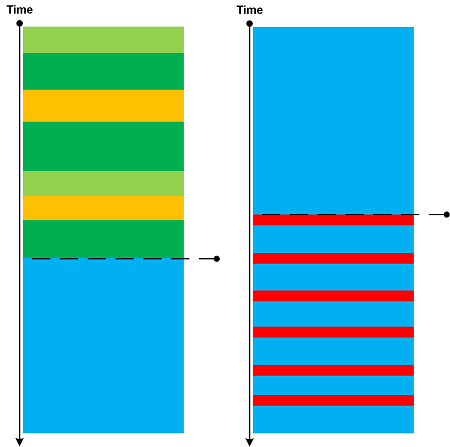## Trace Analysis Patterns (Part 166)

Based on a mathematical analogy with critical points in topology (Morse theory) we introduce Critical Points in trace and log analysis where they signify the change of trace or log “shape” (topological or “geometric” properties) as illustrated in the following diagram:Such a point may be an individual message, its Message Context, or Activity Region.

Critical Points are examples of Intra-Correlation whereas Bifurcation Points are examples of Inter-Correlation.

- Dmitry Vostokov @ DumpAnalysis.org + TraceAnalysis.org -